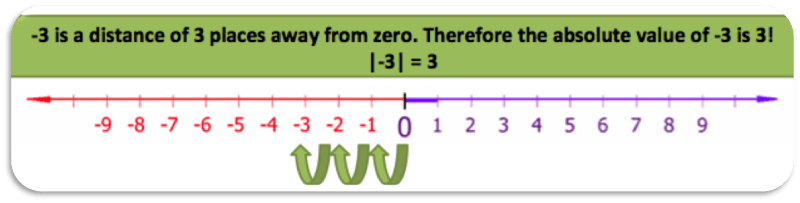# Absolute Value

Absolute value is the distance from zero on a number line. Because it refers to a distance, absolute value is always positive. We use two vertical bars to represent absolute value. If we wanted to take the absolute value of -3, it would look like this: |-3|. This means “find the absolute value of -3” (or the distance that -3 is from zero).

You can find this using one of two ways:

1. What is the positive value for this number?
2. How far from 0 is the number on a number line?

Here is another example. The symbol for absolute value is shown in this equation: |8| = 8 and |-8| = 8. These are read as “The absolute value of 8 equals 8” and “the absolute value of negative 8 equals 8.” These are true because the distance between 0 and 8 on the number line is 8 spaces and the distance between 0 and -8 on the number line is 8 spaces. Distance is always positive. One can never travel a negative distance.

Let’s look at the number line first.Since -3 is 3 units away from 0 on a number line, then the absolute value of -3 is 3. Without a number line, then you take the positive value for -3. So, |-3| = 3

Here are some basic examples:

| 5 | = 5
| -2 | = 2
| 0 | = 0

The distance between a number and zero on the number line is called absolute value.

To solve |-5 + 4|= ?  You first solve what’s inside and get -1 and then find the absolute value, which is 1.  To solve |10| + |-6|= ?  You first find the absolute value of each and then add them 10 + 6 = 16.

## Practice

1)  What is the absolute value of 21?

2)  What is the absolute value of -4?

3)  |-10| = ?

4)  |-3 + 1|= ?

5)  |2 + 3|= ?

6)  |0| = ?

7)  |14 – 20|= ?

8)  |-9 – 2|= ?

9)  |2| + |-18|= ?

(source)